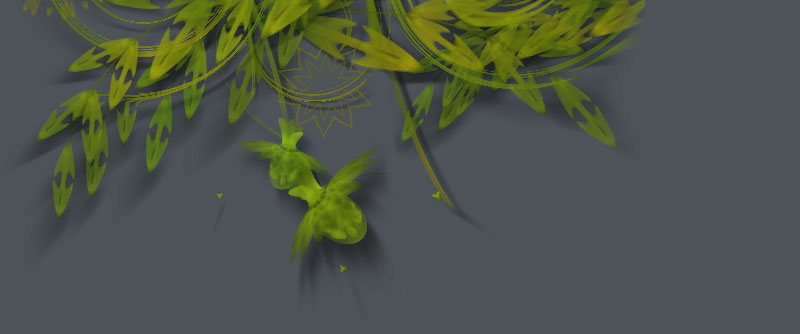### Blines and Circloids

Boids are elements that describe leaderless groups like flocks, herds or schools, according to the algorithm made in 1986 by Craig Reynolds. Boids have no mathematical equations describing their path: they move along individually, and as an emergent group steering clear of each other and moving in the direction everyone else is going.

Boids provide a good a way to describe natural compositions.

Below is an example of curves drawn with a combination of the Boids library and the Cornu spline library, a spiralling curve algorithm by Raph Levien.Source code:

```# You'll need the Boids and Cornu libraries.
boids = ximport("boids")
cornu = ximport("cornu")

size(550, 550)
background(0.1, 0.1, 0.0)
nofill()

flock = boids.flock(10, 0, 0, WIDTH, HEIGHT)

n = 70
for i in range(n):

flock.update(shuffled=False)

# Each flying boid is a point.
points = []
for boid in flock:
points.append((boid.x, boid.y))

# Relativise points for Cornu.
for i in range(len(points)):
x, y = points[i]
x /= 1.0 * WIDTH
y /= 1.0 * HEIGHT
points[i] = (x,y)

t = float(i) / n
stroke(0.9, 0.9, 4*t, 0.6*t)
cornu.drawpath(points, tweaks=0)```

Some older examples, the Circloids, can be viewed here.

Created by Tom De Smedt.